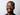Published on: June 07, 2020

# An Introduction to JavaScript Functions

JavaScript

Functions are a powerful tool and super important in JavaScript land. They can be described as a block of statements that let you perform a task. One of the best things about functions is that once you define a function, you can reuse it throughout your code.

## What is a JavaScript Function?

Why don't we start looking at functions via an example. Let's say you want to add five to a number. Here's a way to do it without functions.

``let firstNumber = 1 + 5; // Answer: 6``

Now, lets see an example using a function

``````// This is a function below
return number + 5;
}

Looking at these two examples above your first thought might be that the function required more code to get the same result. That would be a fair thing to say when you only need to do something once, but what if you needed to add five to more numbers? In that case, we can see how a function might be cleaner.

``````function addFive(number) {
return number + 5;
}

In the example above we can see how awesome it is to be able to write something once and reuse it. Functions help you reduce, reuse and recycle your code 🕺🏾. With the above example in mind, let's check out the anatomy of functions.

### How to Call a Function

When you are finished defining your function you can call it by writing its name + parenthesis. Here is an example:

``````function greeting() {
console.log('Hey There');
}

greeting();``````

## The Anatomy of Functions

In the above examples we saw the awesomeness of functions and what they can do, but now why don't we look at how to make them.

Here is our above example again for reference:

``````// This is a function below
return number + 5;
}``````

And here is a nice illustration of what's going on here.1. *Function Keyword: this keyword tells JavaScript that we are trying to define a function.
2. Function Name: this is name of the function and what we use to call the function.
3. Function Parameters: this is the list of variables that you want to use with the function. You can think of it like passing things into the function.
4. Return Keyword: the return keyword is how we pass things back out of our function.

### A Declaration vs An Expression

The above example is what you would call a function declaration. It's a declaration because it's declared using the function keyword. The other major way to define a function is through a function expression. This is when you declare a function like a variable. Here is the same function written as an expression:

``````const addFive = (number) => {
return number + 5;
};``````

So, what's the biggest difference? The biggest difference between the two would be in their hoisting. Function declarations are hoisted, but expressions are not. There's more to hoisting, and here is the MDN page for reference: JavaScript Hoisting.

### Parameters vs Arguments

In the picture above we can see our parameters list. This is the list of things that we want the function to expect. However, when you are using a function and passing those items in, they are called arguments. This concept broke my brain when I was first learning 😂. Now I think about it like this: parameters are empty placeholders to be expected, arguments are full items to be used. Here is a code example:

``````let numberFive = 5;

// Parameters
return number + 5;
}

// Arguments

## Arrow Functions

Arrow functions are a popular way to define functions. The below example shows us what an arrow function looks like in javaScript. They are characterized by the arrow in the syntax.

``````const greeting = () => {
console.log('Hello World');
};``````

### Parameters & Arguments in Arrow Functions

When using arrow functions, if you only have one parameter or argument you don't have to wrap them in parenthesis. The two examples below give the same result.

``````let greetText = 'Hello Text';

const greeting = (greetText) => {
return console.log(greetText);
}; // Answer = "Hello Text"

const greeting = (greetText) => {
return console.log(greetText);
}; // Answer = "Hello Text"``````

### Implicit vs Explicit Return in Arrow Functions

With arrow functions, there are two ways to return: implicit and explicit.

Explicit Return: This method of returning uses the return keyword to return the result from the function.

``````const greetingExplicit = () => {
return 'Hello World';
};

// greetingExplicit returns "Hello World";``````

Implicit Return: This method of returning doesn't use the return keyword, and returns the function result for you when you are working with a one line function body.

``````const greetingImplicit = () => 'Hello World';

// greetingImplicit returns "Hello World";``````

## That's All Folks

As we can see, functions are a powerful tool inn JavaScript 💪🏾. They let us reduce, reuse and recycle our code which makes it easier and cleaner for us as coders! There is still more you can learn about functions, so here is the MDN page if you want to read more in depth about them. JavaScript Functions MDN Page.